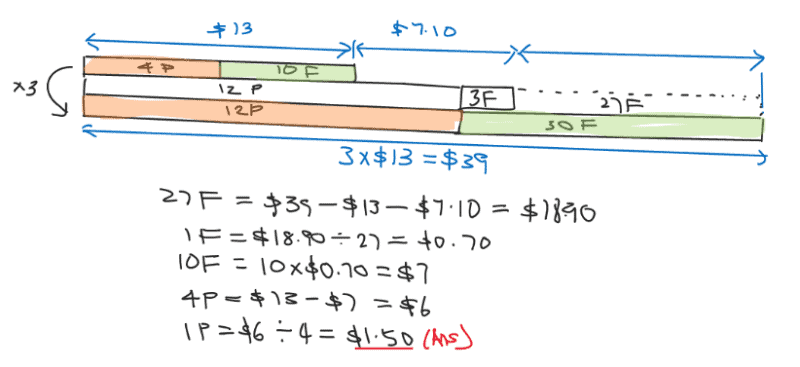# Question

Mary has \$13. She spent all her money on 10 files and 4 pens. If she had bought 3 files and 12 pens instead, she would have a shortage of \$7.10. How much did each pen cost?

Source: Keming Primary0 Replies 2 Likes ✔Accepted Answer

Let’s do it by simultaneous equations.
Let P be the price of a pen.
Let F be the price of a file.

10 F + 4 P = 13
=> F + 0.4P = 1.3
=> F = 1.3 – 0.4P … (1)

3 F + 12 P = 13 + 7.10 = 20.10
=> F + 4P = 20.10/3 = 6.70
=> F = 6.70 – 4P … (2)

Substituting (1) into (2):
1.3 – 0.4P = 6.7 -4P
=> 3.6 P = 5.4
=> P = 5.4/3.6 = 1.50 #

0 Replies 1 Like